Technical Information Site of Power Supply Design

2018.01.11 Transfer Function

# Example of Deviation for a Step-up Converter

DC/DC Converters: Sharing of Transfer Functions among Control Systems

In succession to the previous discussion of step-down converters, this time we present a derivation example for step-up converters. Please refer to the previous section as necessary.

Example of Transfer Function Derivation for Step-up Converters

This time, we shall derive the transfer functions for a step-up converter. But as with a step-down converter, the transfer functions to be derived are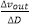and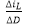. When deriving the transfer functions for step-down converters, the following two steps were taken; and when addressing step-up converters as well, we resort to the same steps for our derivation of the transfer functions.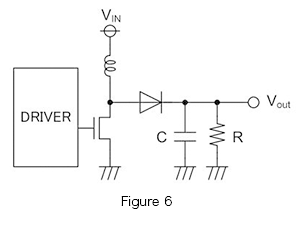●Step 1: Consider the stable states of the system

The procedure is similar to that for the step-down converter. Figure 6 is the basic circuit of a diode-rectified step-up converter. The stable states of the system are the following two states; each is represented by an equation and a graph.

① The coil current does not change over one period
② The capacitor charge amount does not change over one period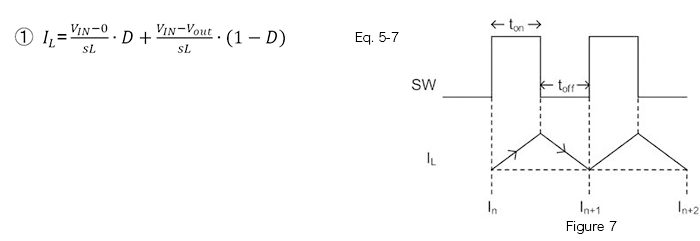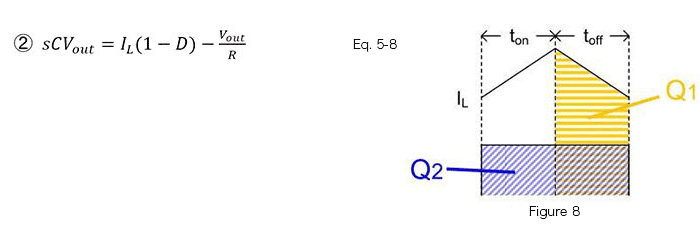●Step 2: Determine change amounts for an external disturbance, and describe the transfer functions

Calculation examples are shown below.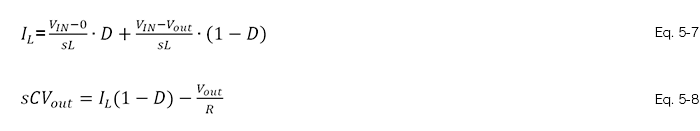Upon substituting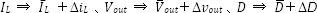in equations 5-7 and 5-8, the following are obtained.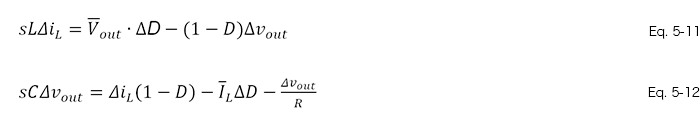Then, taking equations 5-11 and 5-12 to be simultaneous equations and determiningand, we obtain the following.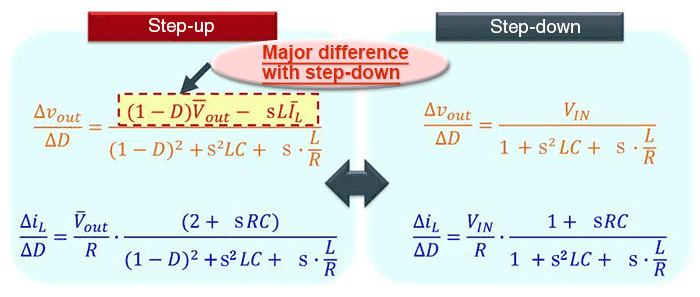For the sake of comparison, the transfer functions have been provided along with those for step-down converters. Of course, the derivation results are different, but what should be noted here is that the same approach as with the step-down mode is used for the step-up mode as well to deriveand.

This website uses cookies.

By continuing to browse this website without changing your web-browser cookie settings, you are agreeing to our use of cookies.